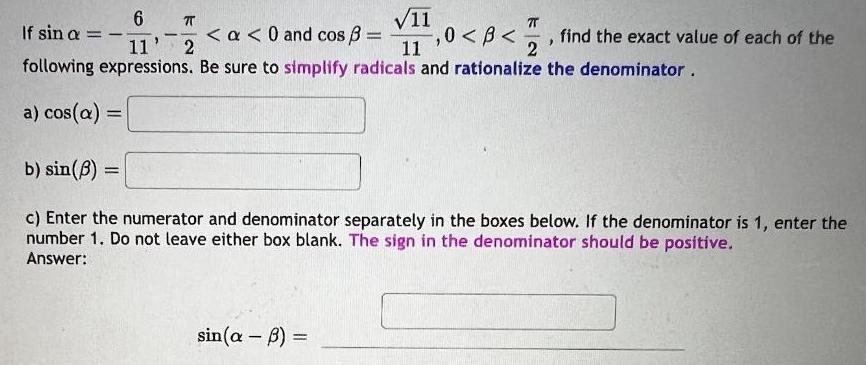Question:

# 11 If sin a 6 TT 11 2 11 2 following expressions Be sure to

Last updated: 7/17/202311 If sin a 6 TT 11 2 11 2 following expressions Be sure to simplify radicals and rationalize the denominator a cos a a 0 and cos 1 0 B find the exact value of each of the b sin 3 c Enter the numerator and denominator separately in the boxes below If the denominator is 1 enter the number 1 Do not leave either box blank The sign in the denominator should be positive Answer sin a B